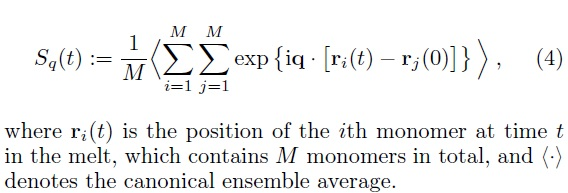# coherent scattering function calculation

Hi LAMMPS users,

I have a question on calculating the coherent scattering function S(q,t) (equation attached). Of course, it is not a problem related to the LAMMPS. However, it bothers me for a long time, since my script dose not give me reasonable results. Therefore, I am wondering whether anybody can send me a sample code for calculating S(q,t)?

Any suggestions/comments are highly appreciate.
All my best,You could write a compute to calculate
this, which in turn would use fix store/state
to store the r(0) positions. See compute msd
for a similar example.

Steve